## Tuesday, January 31, 2017

### January 2017: Common Core Algebra Regents, Part 3

The following are the questions and answers (and commentary) for part of the New York State Algebra Regents exam. If you have any questions or comments (or corrections), please add them in the Comments section.
Part II was posted here.

### January 2017, Algebra I (Common Core), Part III

33. Graph f(x) = | x | and g(x) = -x2 + 6 on the grid below.
Does f(-2) = g(-2)? Use your graph to explain why or why not.

Note that if you explain using substitution instead of graphing, you will not get credit.
The graph looks like this:

So f(-2) = g(-2) because f(-2) = 2 and g(-2) = 2. The two graphs intersect at the point (-2, 2).

34. Two friends went to a restaurant and ordered one plain pizza and two sodas. Their bill totaled \$15.95. Later that day, five friends went to the same restaurant. They ordered three plain pizzas and each person had one soda. Their bill totaled \$45.90.
Write and solve a system of equations to determine the price of one plain pizza.
[Only an algebraic solution can receive full credit.]

Let p = the price of one pizza and let s = the price of one soda. The system of equations would then be:

1p + 2s = 15.95
3p + 5s = 45.90

You can solve this system using elimination by multiplying the first equation by -3, or by substitution if you solve the first equation for p by subtracting 2s from both sides.

Elimination first:

-3p - 6s = -47.85
3p + 5s = 45.90
-s = -1.95
s = 1.95
Plug 1.95 into either equation:
p + 2(1.95) = 15.95
p + 3.90 = 15.95
p =12.05
The price of a pizza is \$12.05.

Substitution:

p = -2s + 15.95
3(-2s + 15.95) + 5s = 45.90
-6s - 47.85 + 5s = 45.90
-s - 47.85 = 45.90
-s = -1.95
s = 1.95
Plug 1.95 into either equation:
p + 2(1.95) = 15.95
p + 3.90 = 15.95
p =12.05
The price of a pizza is \$12.05.

Yes, that is an unusual amount for a pizza, but they didn't want to make it "obvious". \$1.95 is a reasonable price for a soda.

35. Tanya is making homemade greeting cards. The data table below represents the amount she spends in dollars,f(x), in terms of the number of cards she makes, x.

Write a linear function, j(x), that represents the data.
Explain what the slope and y-intercept of j(x) mean in the given context.

You need to find the slope and the y-intercept, which means the rate of change and the initial value.

Pick any two points on the table. Let's say you hate decimals, so you pick (6, 9) and (10, 12). Rate of change is the difference of the y values divided by the difference of the x-values. That is, (12 - 9) / (10 - 6) = 3 / 4 or .75. (You might want to use the decimal for the calculator in the next step.)

Next, you need the y-intercept. Start with y = mx + b and plug in values for x, y and m:

12 = (.75)(10) + b
12 = 7.5 + b
4.5 = b

The function is f(x) = .75x + 4.5

You need to explain the answer in context of the problem. \$4.50 is the initial value, the starting cost for making the cards, and \$0.75 is the cost per card.

36. Alex launched a ball into the air. The height of the ball can be represented by the equation h = -8t2 + 40t + 5, where his the height, in units, and t is the time, in seconds, after the ball was launched. Graph the equation from t = 0 to t= 5 seconds.

State the coordinates of the vertex and explain its meaning in the context of the problem.

The graph will look like this:

Notice that the vertex occurs between 2 and 3, so it must be 2.5 (which is exactly in the middle).

To find the height at t=2.5, substitute into the equation, and enter it into your calculator:
h = -8(2.5)2 + 40(2.5) + 5 = 55
The vertex is (2.5, 55). At 2.5 seconds the ball reaches its highest point, which is 55 feet units in the air.

EDIT: the units are "units" not feet. You don't have to write "units", but if you write "feet" you will lose a point for misidentifying the units.
The problem is in neither feet nor meters. A gravity problem dealing using feet would usually start with -16t2. A problem with meters would usually start with a leading coefficient of -4.9 or -5, depending upon the desired precision.

End of Part III

How did you do?
Comments, questions, corrections and concerns are all welcome.
Typos happen.

### Ken-Do #13: German

(Click on the comic if you can't see the full image.)Tell me about it! The first 1100+ comics are the hardest!

The wurst thing about this: at first, I thought, I can't do this. But then the song came into my head and the words wrote seemed to write themselves ... until I tried writing them down and I realized I didn't have as much as I thought. Do you know why?

Because my muse is a brat!

Come back often for more funny math and geeky comics.## Monday, January 30, 2017

### January 2017: Common Core Algebra Regents, Part 2

The following are the questions and answers (and commentary) for part of the New York State Algebra Regents exam. If you have any questions or comments (or corrections), please add them in the Comments section.

### January 2017, Algebra I (Common Core), Part II

25. In attempting to solve the system of equations y = 3x - 2 and 6x - 2y = 4, John graphed the two equations on his graphing calculator. Because he saw only one line, John wrote that the answer to the system is the empty set. Is he correct? Explain your answer.

John is not correct. The answer is not the empty set. The answer is that the two lines are coincident (they overlap each other), so there are an infinite number of points that solve this system of equations.

Proof: 6x - 2y = 4
-2y = -6x + 4
y = 3x - 2, which is the other line.
The two lines are coincidental lines.

The empty set would mean that the lines never intersected; i.e., they are parallel and have no points in common.

26. A typical marathon is 26.2 miles. Allan averages 12 kilometers per hour when running in marathons. Determine how long it would take Allan to complete a marathon, to the nearest tenth of an hour. Justify your answer.

"Justify" means that you need to show your work or offer some kind of proof.
This problem is about unit conversion, and the conversion you need is in the back of the book: 1 mile = 1.60943 km
26.2 miles = 26.2(1.60943 km/mi) = 42.164708 km
42.164708 km / (12 km/hr) = 3.5137256666....
Which is 3.5 to the nearest tenth of an hour.

27. Solve the inequality below: 1.8 - 0.4y > 2.2 - 2y

Solve inequalities like you solve equations, but be mindful of dividing by negative numbers.

1.8 - 0.4y > 2.2 - 2y
-0.4y > 0.4 - 2y
-0.4y > 0.4 - 2y
1.6y > 0.4
y > 0.25

Unusual that they didn't multiply or divide by a negative, but watch out for it.

28. Jakob is working on his math homework. He decides that the sum of the expression 1/3 + 6*sqrt(5)/7 must be rational because it is a fraction. Is Jakob correct? Explain your reasoning.

Jakob is not correct. For a fraction to be rational, both the numerator and the denominator must be rational numbers. Six radical Five is an irrational number, and dividing it by 7 doesn't make it rational.

Finally, the sum of any rational number (e.g., 1/3) and an irrational number is always irrational.

29. Graph the inequality y > 2x - 5 on the set of axes below. State the coordinates of a point in its solution.

The graph will be a broken line with a slope of 2 and a y-intercept of -5 that is shaded ABOVE the line.
If you don't remember where to shade, here is the simple test: take the point (0, 0) and substitute it into the inequality. If the result a true statement? Is 0 > 2(0) - 5? Yes, 0 > -5, so that point is in the shaded region. Because that point is above the line, you will shade above the line. Like this:

Remember to state ANY point in the solution. (0, 0) would work. Or (-10, 0). Or (0, 10). Any point in the shaded section that is NOT on the line. The line is NOT part of the solution because it is broken.

30. Sandy programmed a website's checkout process with an equation to calculate the amount customers will be charged when they download songs. The website offers a discount. If one song is bought at the full price of \$1.29, then each additional song is \$.99. State an equation that represents the cost, C, when s songs are downloaded.
Sandy figured she would be charged \$52.77 for 52 songs. Is this the correct amount? Justify your answer.

The linear equation that models this problem is (Note that the variables are up to you): C = 1.29 + .99(n - 1)
You pay \$1.29 for the first song. If you buy n song, you will pay 99 cents for n - 1 of them.

To find the cost of 52 songs, substitute 52 for n: C = 1.29 + .99(52 - 1) = 51.78
Sandy is incorrect.

Not that they asked this, but it looks like her mistake is that she calculated C = 1.29 + .99(52), without subtracting 1 first.

31. A family is traveling from their home to a vacation resort hotel. The table below shows their distance from home as a function of time.

 Time (hrs) 0 2 5 7 Distance (mi) 0 140 375 480

Determine the average rate of change between hour 2 and hour 7, including units.

Average rate of change between points (2, 140) and (7, 480) is the difference of the y values divided by the difference of the x values:
(480 - 140) / (7 - 2) = 340 / 5 = 68 mi / hr.

Note that the question said to include units, so you better include the units or you will lose a point!

32. Nora says that the graph of a circle is a function because she can trace the whole graph without picking up her pencil.
Mia says that a circle graph is not a function because multiple values of x map to the same y-value.

Neither one is correct. Nora is wrong because not lifting a pencil is not the definition of a function. Piecewise functions sometimes require you to lift your pencil. Mia is wrong because she has it backwards: there are multiple values of y for the same x, which is the vertical line test. What Mia described would be a horizontal line test, but there is no such thing for functions. You are allowed to have multiple values of x map to the same value of y. (For example: the absolute value function repeats y values for more than one x.)

End of Part II

How did you do?
Comments, questions, corrections and concerns are all welcome.
Typos happen.

## Friday, January 27, 2017

### Coincidence

(Click on the comic if you can't see the full image.)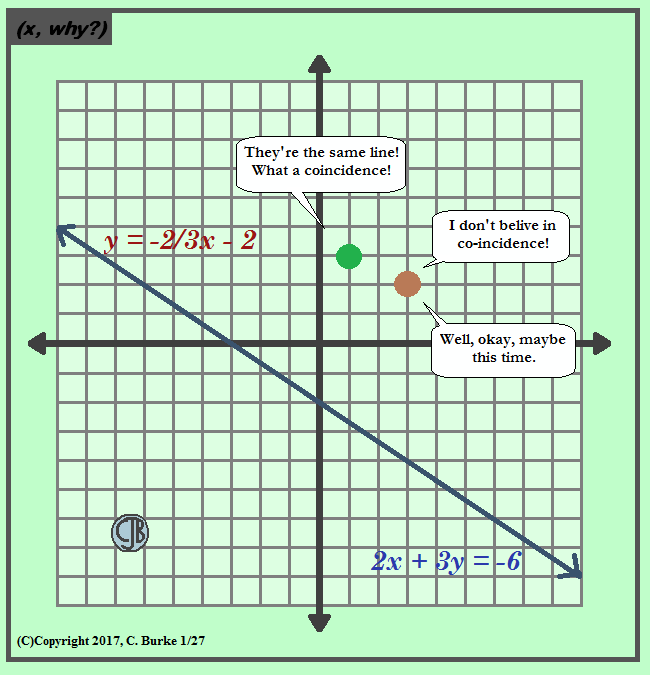Is this tagline a coincidence or was it preplanned?

Is it coincidence that the date is 01-27-2017, using the same number twice is different orders?

Come back often for more funny math and geeky comics.## Friday, January 20, 2017

### (x, why?) Mini: Math Laws

(Click on the comic if you can't see the full image.)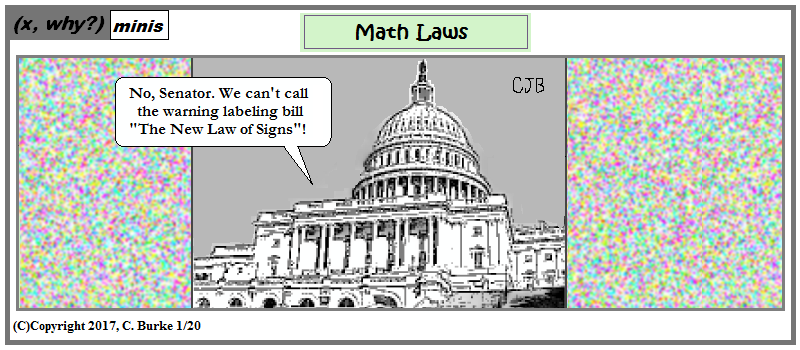The biggest irony here is that I started doing 'minis' because I didn't have time for full comics. Yet I haven't had a chance to do a mini in over three months!

The last time I used this image, the national debt ceiling was just raised to \$14 trillion. My, how times have changed!

Come back often for more funny math and geeky comics.## Friday, January 13, 2017

(Click on the comic if you can't see the full image.)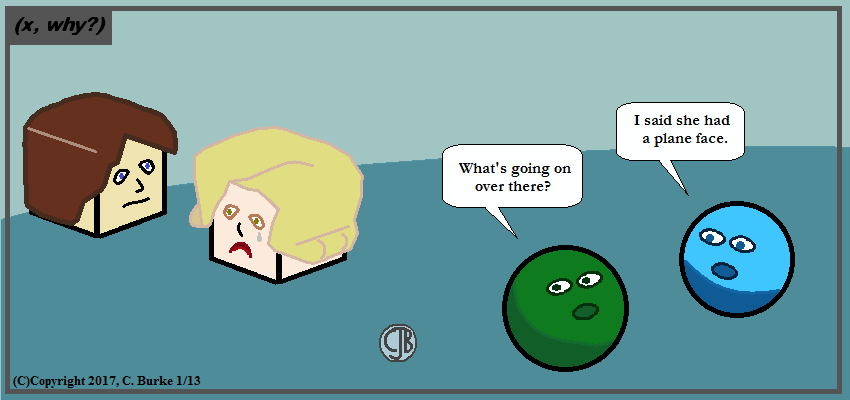Kind of like what his face would be after she slaps him to the ground!

(I wonder how I haven't used this in the past 9+ years!)

Come back often for more funny math and geeky comics.## Wednesday, January 11, 2017

### Cinnamon Rolls

(Click on the comic if you can't see the full image.)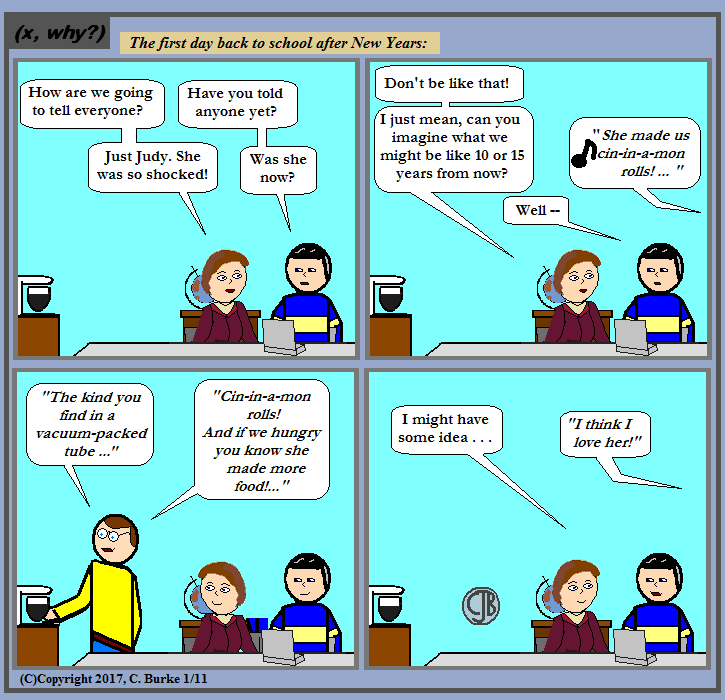Shocked! Shocked, I tell you!

Regular readers will know why Judy probably wasn't as shocked as she might have appeared.

So this should've been up sometime last week, but, well, you know. Plus the song has been on the back burner since a #hashtag game on Twitter a couple months back.

If you don't know what they're Michele and Wayne are talking about, you need to click back to New Years. Okay, here's the link.

Come back often for more funny math and geeky comics.## Thursday, January 05, 2017

### Theoretical Weight

(Click on the comic if you can't see the full image.)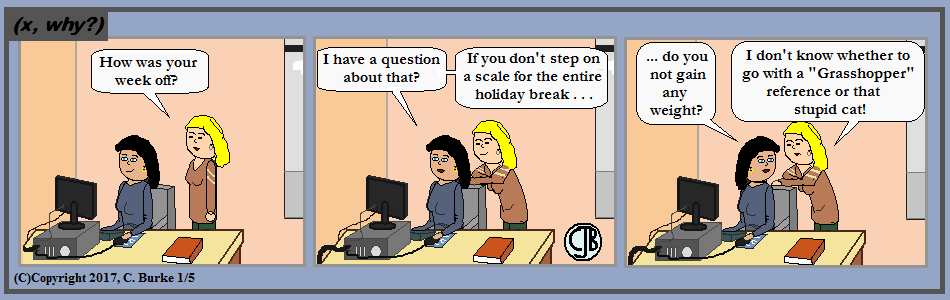I wouldn't say Schrödinger's cat was stupid, but it did get stuck in that box.

Maybe you only gained half the weight?

The dimensions of this comic are closer to what other webcomics seem to use when they follow the "strip" format. I'm not sure that I like it. It might look good in a book, but who would buy a print book of (x, why?)? On the other hand, I'm sure it's seen on many screens, so dimensions closer to a Golden Ratio might be better.

Come back often for more funny math and geeky comics.## Sunday, January 01, 2017

### Happy New Year 2017: Dependent Events

(Click on the comic if you can't see the full image.)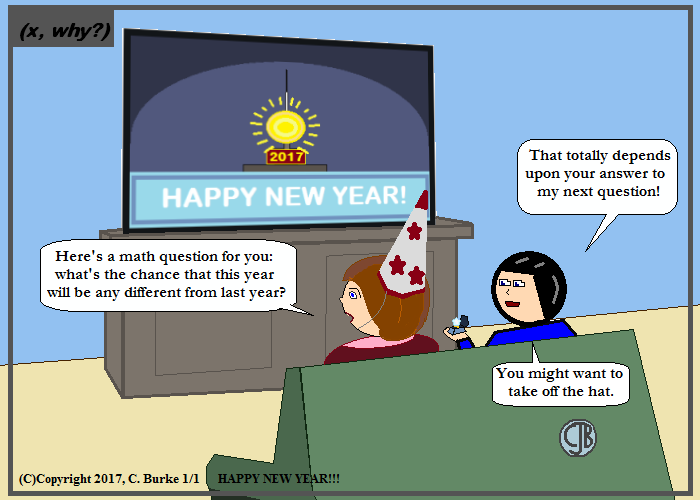Two events are dependent if the outcome of the first affects the outcome of the second.

Safe to say, in this case, this year's going to be different either way. So 100%.

Also, in either case, I don't think I'll be drawing my characters from this direction again any time soon.

Come back often for more funny math and geeky comics.